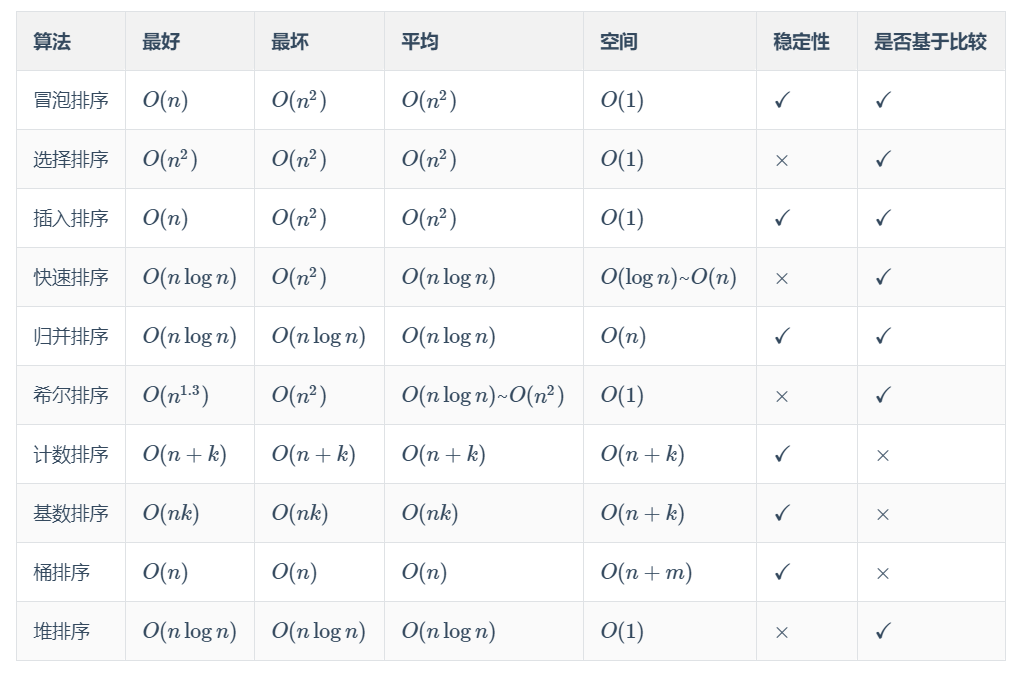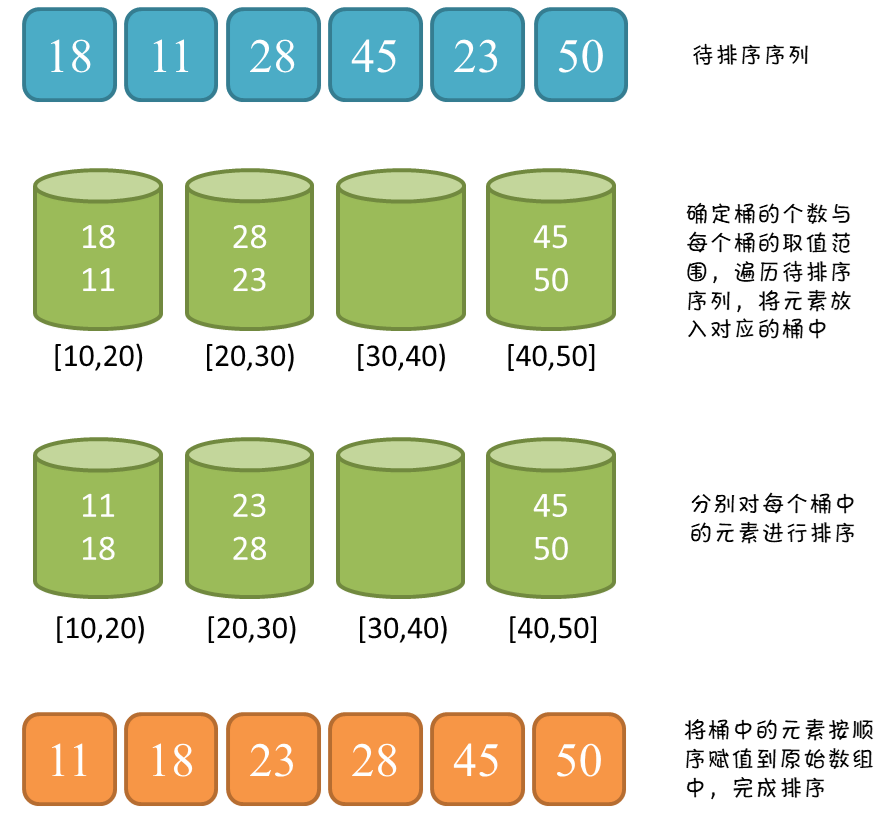# 数据结构之十大经典排序算法汇总

#### 性能汇总

• 若显示有问题，则可参考下方汇总图#### 1、冒泡排序（Bubble Sort）

①、基本思想：两个数比较大小，较大的数下沉，较小的数冒起来。

②、算法描述

③、代码实现

`````` public static int[] bubbleSort(int[] array) {
if (array.length == 0)
return array;
for (int i = 0; i < array.length-1; i++)
for (int j = 0; j < array.length - 1 - i; j++)
if (array[j + 1] < array[j]) {
int temp = array[j + 1];
array[j + 1] = array[j];
array[j] = temp;
}
return array;
}``````

#### 2、选择排序（Selection Sort）

①、基本思想：选择排序(Selection-sort)是一种简单直观的排序算法。它的工作原理：首先在未排序序列中找到最小（大）元素，存放到排序序列的起始位置，然后，再从剩余未排序元素中继续寻找最小（大）元素，然后放到已排序序列的末尾。以此类推，直到所有元素均排序完毕。

②、算法描述：（n个记录的直接选择排序可经过n-1趟直接选择排序得到有序结果。）

n-1趟结束，数组有序化了。

③、代码实现

``````public static int[] selectionSort(int[] array) {
if (array.length == 0)
return array;
for (int i = 0; i < array.length; i++) {
int minIndex = i;
for (int j = i; j < array.length; j++) {
if (array[j] < array[minIndex]) //找到最小的数
minIndex = j; //将最小数的索引保存
}
int temp = array[minIndex];
array[minIndex] = array[i];
array[i] = temp;
}
return array;
}``````

#### 3、插入排序（Insertion Sort）

①、基本思想：在要排序的一组数中，假定前n-1个数已经排好序，现在将第n个数插到前面的有序数列中，使得这n个数也是排好顺序的。如此反复循环，直到全部排好顺序。

②、算法描述

③、代码实现

``````public static int[] insertionSort(int[] array) {
if (array.length == 0)
return array;
int current;
for (int i = 0; i < array.length - 1; i++) {
current = array[i + 1];
int preIndex = i;
while (preIndex >= 0 && current < array[preIndex]) {
array[preIndex + 1] = array[preIndex];
preIndex--;
}
array[preIndex + 1] = current;
}
return array;
}``````

#### 4、希尔排序（Shell Sort）

①、基本思想：希尔排序也是一种插入排序，它是简单插入排序经过改进之后的一个更高效的版本，也称为缩小增量排序，同时该算法是冲破O(n2）的第一批算法之一。它与插入排序的不同之处在于，它会优先比较距离较远的元素。

②、算法描述

③、代码实现

`````` public static int[] ShellSort(int[] array) {
int len = array.length;
int temp, gap = len / 2;
while (gap > 0) {
for (int i = gap; i < len; i++) {
temp = array[i];
int preIndex = i - gap;
while (preIndex >= 0 && array[preIndex] > temp) {
array[preIndex + gap] = array[preIndex];
preIndex -= gap;
}
array[preIndex + gap] = temp;
}
gap /= 2;
}
return array;
}``````

#### 5、归并排序（Merge Sort）

①、基本思想

②、算法描述

③、代码实现

``````/**
* 归并排序
* @param array
* @return
*/
public static int[] MergeSort(int[] array) {
if (array.length < 2) return array;
int mid = array.length / 2;
int[] left = Arrays.copyOfRange(array, 0, mid);
int[] right = Arrays.copyOfRange(array, mid, array.length);
return merge(MergeSort(left), MergeSort(right));
}
/**
* 归并排序——将两段排序好的数组结合成一个排序数组
* @param left
* @param right
* @return
*/
public static int[] merge(int[] left, int[] right) {
int[] result = new int[left.length + right.length];
for (int index = 0, i = 0, j = 0; index < result.length; index++) {
if (i >= left.length)
result[index] = right[j++];
else if (j >= right.length)
result[index] = left[i++];
else if (left[i] > right[j])
result[index] = right[j++];
else
result[index] = left[i++];
}
return result;
}``````

#### 6、快速排序（Quick Sort）

①、基本思想（分治）：

②、算法描述：快速排序使用分治法来把一个串（list）分为两个子串（sub-lists）。具体算法描述如下：

③、代码实现

``````  public static void quickSort(int[] arr, int L, int R) {
int i = L;
int j = R;
//支点
int pivot = arr[(L + R) / 2];
//左右两端进行扫描，只要两端还没有交替，就一直扫描
while (i <= j) {
//寻找直到比支点大的数
while (pivot > arr[i])
i++;
//寻找直到比支点小的数
while (pivot < arr[j])
j--;
//此时已经分别找到了比支点小的数(右边)、比支点大的数(左边)，它们进行交换
if (i <= j) {
int temp = arr[i];
arr[i] = arr[j];
arr[j] = temp;
i++;
j--;
}
}
//上面一个while保证了第一趟排序支点的左边比支点小，支点的右边比支点大了。

//“左边”再做排序，直到左边剩下一个数(递归出口)
if (L < j)
quickSort(arr, L, j);
//“右边”再做排序，直到右边剩下一个数(递归出口)
if (i < R)
quickSort(arr, i, R);
}``````

#### 7、堆排序（Heap Sort）

①、基本思想：堆排序（Heapsort）是指利用堆这种数据结构所设计的一种排序算法。堆积是一个近似完全二叉树的结构，并同时满足堆积的性质：即子结点的键值或索引总是小于（或者大于）它的父节点。

②、算法描述

③、代码实现

``````static int len;
/**
* 堆排序算法
*
* @param array
* @return
*/
public static int[] HeapSort(int[] array) {
len = array.length;
if (len < 1) return array;
//1.构建一个最大堆
buildMaxHeap(array);
//2.循环将堆首位（最大值）与末位交换，然后在重新调整最大堆
while (len > 0) {
swap(array, 0, len - 1);
len--;
}
return array;
}
/**
* 建立最大堆
*
* @param array
*/
public static void buildMaxHeap(int[] array) {
//从最后一个非叶子节点开始向上构造最大堆
for (int i = (len/2 - 1); i >= 0; i--) {
}
}
/**
* 调整使之成为最大堆
*
* @param array
* @param i
*/
public static void adjustHeap(int[] array, int i) {
int maxIndex = i;
//如果有左子树，且左子树大于父节点，则将最大指针指向左子树
if (i * 2 < len && array[i * 2] > array[maxIndex])
maxIndex = i * 2;
//如果有右子树，且右子树大于父节点，则将最大指针指向右子树
if (i * 2 + 1 < len && array[i * 2 + 1] > array[maxIndex])
maxIndex = i * 2 + 1;
//如果父节点不是最大值，则将父节点与最大值交换，并且递归调整与父节点交换的位置。
if (maxIndex != i) {
swap(array, maxIndex, i);
}
}``````

#### 8、计数排序（Counting Sort）

①、基本思想：计数排序不是基于比较的排序算法，其核心在于将输入的数据值转化为键存储在额外开辟的数组空间中。 作为一种线性时间复杂度的排序，计数排序要求输入的数据必须是有确定范围的整数。

②、算法描述

③、代码实现

``````
public static int[] CountingSort(int[] array) {
if (array.length == 0) return array;
int bias, min = array, max = array;
for (int i = 1; i < array.length; i++) {
if (array[i] > max)
max = array[i];
if (array[i] < min)
min = array[i];
}
bias = 0 - min;
int[] bucket = new int[max - min + 1];
Arrays.fill(bucket, 0);
for (int i = 0; i < array.length; i++) {
bucket[array[i] + bias]++;
}
int index = 0, i = 0;
while (index < array.length) {
if (bucket[i] != 0) {
array[index] = i - bias;
bucket[i]--;
index++;
} else
i++;
}
return array;
}``````

#### 9、桶排序（Bucket Sort）

①、基本思想

②、算法描述

③、图片演示④、代码实现

``````public static void bucketSort(int[] arr){

// 计算最大值与最小值
int max = Integer.MIN_VALUE;
int min = Integer.MAX_VALUE;
for(int i = 0; i < arr.length; i++){
max = Math.max(max, arr[i]);
min = Math.min(min, arr[i]);
}

// 计算桶的数量
int bucketNum = (max - min) / arr.length + 1;
ArrayList<ArrayList<Integer>> bucketArr = new ArrayList<>(bucketNum);
for(int i = 0; i < bucketNum; i++){
}

// 将每个元素放入桶
for(int i = 0; i < arr.length; i++){
int num = (arr[i] - min) / (arr.length);
}

// 对每个桶进行排序
for(int i = 0; i < bucketArr.size(); i++){
Collections.sort(bucketArr.get(i));
}

// 将桶中的元素赋值到原序列
int index = 0;
for(int i = 0; i < bucketArr.size(); i++){
for(int j = 0; j < bucketArr.get(i).size(); j++){
arr[index++] = bucketArr.get(i).get(j);
}
}
}``````

①、基本思想

②、算法描述

③、代码实现

`````` public static void radixSort(int[] arrays) {

if (arrays == null || arrays.length < 2)
return arrays;
// 1.先算出最大数的位数；
int max = arrays;
for (int i = 1; i < arrays.length; i++) {
max = Math.max(max, arrays[i]);
}
//需要遍历的次数由数组最大值的位数来决定
for (int i = 1; max / i > 0; i = i * 10) {

int[][] buckets = new int[arrays.length];

//获取每一位数字(个、十、百、千位...分配到桶子里)
for (int j = 0; j < arrays.length; j++) {

int num = (arrays[j] / i) % 10;

//将其放入桶子里
buckets[j][num] = arrays[j];
}

//回收桶子里的元素
int k = 0;

//有10个桶子
for (int j = 0; j < 10; j++) {
//对每个桶子里的元素进行回收
for (int l = 0; l < arrays.length ; l++) {
//如果桶子里面有元素就回收(数据初始化会为0)
if (buckets[l][j] != 0) {
arrays[k++] = buckets[l][j];

}

}

}

}
}``````

目录